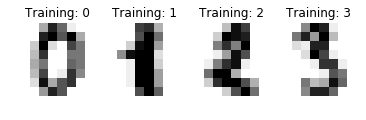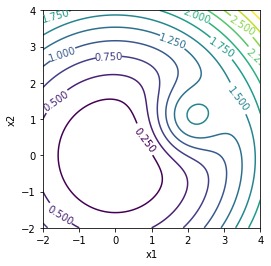# Homework 4¶

## Homework Submission Workflow¶

When you submit your work, follow the instructions on the submission workflow page scrupulously for full credit.

Important: Failure to do any of the following will result in lost points:

• Submit one PDF file and one notebook per group

• Enter all the group members for the PDF submission in Gradescope, not just in your documents. Look at this Piazza Post if you don't know how to do this

• Match each answer (not question!) with the appropriate page in Gradescope

• Avoid large blank spaces in your PDF file

## Part 1: Nearest Neighbors¶

Homework 3 explored a possible implementation of $k$-Nearest-Neighbors ($k$-NN) predictors. That implementation assumed that no work at all is done at training time, other than storing the entire data set $T$. In this part of this assignment, you will be using the $k$-NN classifier in scikit-learn instead. This implementation sets up a suitable data structure at training time in order to speed up inference.

We use Euclidean distance in all cases in this part.

To get you started, the following code computes the risk of a $k$-NN classifier, assuming that the labels $y\in Y$ are represented by integers. The classifier is trained on set T and the risk is computed on set S, both stored as dictionaries with keys x and y, as usual. The call to h.fit sets up the data structure, so you can view that call as some sort of "training."

In :
import numpy as np
from sklearn import neighbors

def risk(k, T, S):
h = neighbors.KNeighborsClassifier(k)
h.fit(T['x'], T['y'])
y = h.predict(S['x'])
return 1 - np.sum(S['y'] == y) / len(S['y'])


### Problem 1.1¶

What loss does the risk function above use?

### Problem 1.2¶

What is the value of

risk(1, T, T)



regardless of what training set $T$ is used, assuming that all data points $\mathbf{x}$ in $T$ are distinct? Justify your answer with brief and clear text that involves this last assumption.

### Problem 1.3¶

risk(k, T, T)



with $k > 1$? Explain briefly and clearly.

### Problem 1.4¶

Let us now remove the assumption that all data points $\mathbf{x}$ in $T$ are distinct. Describe briefly and clearly what would have to happen for the value of

risk(1, T, T)



to have a different value from what you gave in your answer to problem 1.2.

### Data¶

The following code, adapted from the scikit-learn tutorial, loads a miniature version of the MNIST handwritten digit recognition dataset used to train and evaluate classifiers. The data is stored in a dictionary data with keys 'x' and 'y', as usual.

The code below also displays four of the images as an example. The images are very small, $8\times 8$ pixels, and the code flattens them into vectors of $64$ numbers. Each vector is a row of data['x'], and corresponds to a datapoint $\mathbf{x}$. The corresponding labels $y$ are integers between $0$ and $9$ and are stored in data['y'].

In :
%matplotlib inline

from sklearn import datasets
import matplotlib.pyplot as plt
images_and_labels = list(zip(digits.images, digits.target))
for index, (image, label) in enumerate(images_and_labels[:4]):
plt.subplot(2, 4, index + 1)
plt.axis('off')
plt.imshow(image, cmap=plt.cm.gray_r, interpolation='nearest')
plt.title('Training: %i' % label)
plt.show()
data = {'x': digits.images.reshape((len(digits.images), -1)), 'y': digits.target}### Some Useful Code¶

We will look at plots of risk for different values of $k$, and we will compute risks for different random splits of the data into training and test set. Computing these plots takes some time, so it's useful to have an indicator of progress, just to know where we are with the computation. The following function is provided for your convenience. It is called once before the computation starts, with argument p equal to the number of iterations in the computation. Then it's called once per iteration. It prints a message every 10% of the way.

In :
from math import floor
def progress(p=0):
try:
progress.initialized = progress.initialized
except AttributeError:
progress.initialized = True
progress.count, progress.last, progress.decade = 0, 0, 0
if  p > 0:
progress.count, progress.last, progress.decade = 0, p, 0
else:
progress.count += 1
decade = floor(10 * progress.count / progress.last)
print(10 * decade, '%', sep='', end=' ')
print()


A function to display the risk plots with error bars superimposed is also provided below. Use either progress or showRisk, or both, as you deem convenient.

In :
def showRisk(kValues, mean, std):
plt.figure()
ax = plt.axes([0, 0, 1, 1])
plt.errorbar(kValues, mean, yerr=std, fmt='-o', lw=2,
capsize=5, ecolor='orange')
ax.set_xticks(kValues)
plt.xlabel('k')
plt.ylabel('Risk (Percent)')
plt.show()


### Problem 1.5¶

Write code that repeats the following computation $30$ times: Split the data into a training set T that contains about 3/4 of the data, selected at random, and a test set S that contains the remaining data. For each split, compute the

risk(k, T, S)



for all odd values of $k$ between $1$ and $21$ inclusive (we consider odd values only so we never have ties). Plot the risk values with error bars. The half-length of the bar for value $k$ is the standard deviation of the risk values over the 30 trials for that value of $k$.

Show your code and the resulting plot, with axes appropriately labeled (showRisk does this for you), and report the mean risk for $k=1$, as a percentage (not a fraction) and with two decimal digits after the period.

#### Programming Notes¶

• It is important to loop over $k$ inside the loop over the trials. In this way, the $30$ splits are the same splits for every $k$, for a fair comparison.

• Do not count on the data being stored in a random order: They are not. To obtain a random permutation of a set of integers, you can use the function numpy.random.permutation.

• It is simplest to first store all risk values in an array, and then use numpy.mean and numpy.std to compute mean and standard deviation.

• Progress indicators are not required, but you may want to use progress anyway, as this is a lengthy calculation.

### Problem 1.6¶

Your plot of the mean risk should by-and-large increases with $k$, except possibly for fluctuations that are small relative to the uncertainty encoded by the error bars. Because of this, $k=1$ is about as good as it gets. This may surprise you, given our class discussion about overfitting in the context of nearest-neighbor prediction. This problem explores why this may be the case for this data set.

For a particular data point $\mathbf{x}$ in the whole data set data, let $d_s$ be the distance between $\mathbf{x}$ and the nearest data point $\mathbf{x}_s$ in data, other than $\mathbf{x}$ itself, that has the same label as $\mathbf{x}$. Furthermore, let $d_d$ be the distance between $\mathbf{x}$ and the nearest data point $\mathbf{x}_d$ in data whose label is different from that of $\mathbf{x}$.

Let us define the margin of $\mathbf{x}$ to be the difference

$$\mu(\mathbf{x}) = d_d - d_s \;.$$

Is a $1$-NN classifier likely to do better when most margins are positive or negative? Justify your answer briefly and clearly.

### Problem 1.7¶

Write code to compute a 100-bin histogram of the margin $\mu(\mathbf{x})$ of the data in data. Show your code and the resulting plot. Your histogram should have properly labeled axes. The values in the histogram should add up to the number of data points, not to $1$. Also report the percentage (not fraction) of points with a negative margin. Display three decimal digits after the period.

## Part 2: Differentiation¶

Let

$$f(\mathbf{x}) = \frac{x_1^2 + x_2^2}{10} + e^{-(\mathbf{x} - \mathbf{c})^T(\mathbf{x} - \mathbf{c})}$$

where

$$\mathbf{x} = \left[\begin{array}{c}x_1\\x_2\end{array}\right]\;\;\;\;\;\text{and}\;\;\;\;\; \mathbf{c} = \left[\begin{array}{c}2\\1\end{array}\right]\;.$$

A labeled contour plot of $f(\mathbf{x})$ with $12$ contours is shown below. The function f(x) computes samples of $f$ on a grid of $100\times 100$ equi-spaced samples in the square $-2 \leq x_1, x_2 \leq 4$.

In :
import numpy as np
import matplotlib.pyplot as plt

def showContours(X, Y):
fig, ax = plt.subplots()
cs = ax.contour(X[:, :, 0], X[:, :, 1], Y, 12)
ax.clabel(cs, inline=1, fontsize=10)
plt.xlabel('x1')
plt.ylabel('x2')
plt.axis('image')
plt.show()

n, mn, mx = 100, -2, 4
x = np.linspace(mn, mx, n)
X = np.dstack(np.meshgrid(x, x))

def inner(x, y):
return x[:, :, 0] ** 2 + y[:, :, 1] ** 2

c, scale = [2, 1], 10

def f(x):
d = np.zeros(x.shape)
for i in range(2):
d[:, :, i] = x[:, :, i] - c[i]
y = inner(x, x) / scale + np.exp(-inner(d, d))
return y

Y = f(X)
showContours(X, Y)### Problem 2.1¶

Write mathematical formulas for the gradient $\nabla f(\mathbf{x})$ and the Hessian $H_f(\mathbf{x})$ of $f$. If you are not sure of your result, show your calculations.

A correct answer gets you full credit, but a compact one leads to simpler code later on.

### Problem 2.2¶

Use your formulas to write code that draws a labeled contour plots of the norm of the gradient of $f$ on a grid of $100\times 100$ equi-spaced samples in the square $-2 \leq x_1, x_2 \leq 4$. Show your code and the resulting plot.

### Problem 2.3¶

Use your formulas to write code that draws an image whose color depends on the signs of the eigenvalues of $H_f(\mathbf{x})$ on a grid of $100\times 100$ equi-spaced samples in the square $-2 \leq x_1, x_2 \leq 4$. Show your code and the resulting plot.

#### Programming Notes¶

Suppose that your code computes an array Lambda with shape (100, 100, 2) so that Lambda[i, j, :] contains the two eigenvalues at grid point $(i, j)$. Then, the function below displays the required image and prints out the meaning of the colors.

In :
def showLambdas(Lambda):
L0 = Lambda[:, :, 0] > 0
L1 = Lambda[:, :, 1] > 0
sign = 0.5 * np.ones(Lambda.shape[:2])
sign[L0 & L1] = 1
sign[(~L0) & (~L1)] = 0

fig, ax = plt.subplots()
ax.imshow(sign, origin='lower')
plt.axis('off')
plt.show()
print('Mapping from colors to signs of the eigenvalues:')
print('Yellow: both positive; Green: mixed; Black: both negative')


### Problem 2.4¶

How many stationary points, and of what types (maximum, minimum, saddle) does $f$ plausibly have in the region of the plot? Justify your answer.

#### Remarks¶

The system of two equations

$$\nabla f(\mathbf{x}) = \mathbf{0}$$

cannot be solved in closed form, so it would be difficult to prove your answer. You may be able to give a plausible answer to the question by reasoning about how the function $f$ is built (look at the code for it) and/or examining the contour plot of the gradient magnitude.

Code somehow like the following may help as well, where G is the array with the values of the gradient magnitude:

fig, ax = plt.subplots()
ax.imshow(G < 0.1, origin='lower')